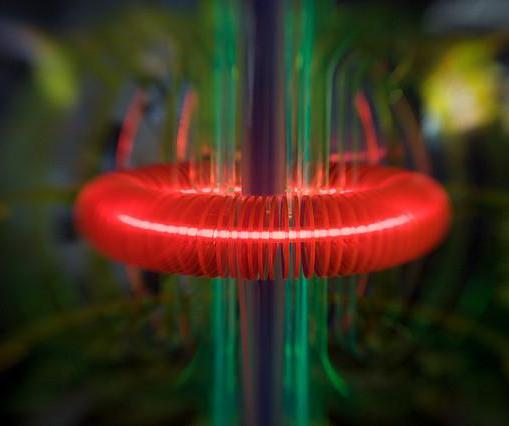What Are the Laws of Energy?

Ray Hawk
Ray Hawk

The laws of energy that govern interactions between matter and energy, such as the transfer of heat from one body to another in the physical universe, are most fundamentally defined by the three laws of thermodynamics and Albert Einstein's discovery of his special and general theories of relativity. Physics itself is built upon these laws, as well as the basic three laws of motion defined by Isaac Newton and first published in 1687, which explain the interaction of all matter. The field of quantum mechanics which began to emerge in the early 20th century also clarified special circumstances for the laws of energy at a sub-atomic scale, upon which much of modern civilization as of 2011 is founded.The laws of energy opened the door for research into fusion reactions and nuclear power.

One of the fundamental principles of the laws of energy made clear by the first law of thermodynamics is that energy is neither created nor destroyed. All forms of energy such as light or sound energy can be changed into other forms, and this was first revealed in the mid-1800s by the work of James Joule, a pioneering English physicist after which the basic unit of energy, the joule, was named. After ten years of thinking about the nature of the relationship between matter and energy, Albert Einstein published his famous formula in 1905 of E=MC2, that stated that both matter and energy were versions of the same thing and could be changed into one another as well. Since the equation states that energy (E) equals mass (M) times the speed of light squared (C2), it was actually stating that, if you had enough energy, you could convert it into mass, and, if you accelerated mass enough, you could convert it into energy.

The second law of thermodynamics defined the laws of energy by stating that, in any activity where energy was used, its potential diminished, or it became less and less available for further work. This reflected the principle of entropy and explained where energy went when heat or light escaped into the surroundings, which had puzzled humanity for centuries. Entropy is the idea that high levels of concentrated energy, such as that in fuel before it is burned, eventually spread out into space as waste heat and cannot be recovered. It was in harmony with the first law of thermodynamics because energy was not being destroyed, but access to it was lost.

The third law of thermodynamics was clarified in 1906 by research conducted by Walther Nernst, a German chemist. It revealed that it was impossible to create a region of space or matter where zero energy existed, which would cool the region to the lowest possible temperature of absolute zero. This supported the first and second laws of thermodynamics in that energy would always be available in space or matter to some degree, even if it could not be harnessed for useful work.

Einstein's updates on our understanding of the laws of energy made many modern technologies possible, such as nuclear power. As well, Newton's laws of motion showed scientists and engineers how to harness the relationship between matter and energy to generate the force and trajectory necessary to put satellites into orbit or send space probes to nearby planets. Quantum mechanics has contributed to the understanding of how energy is used and transferred to create technology such as lasers, transistors that are the foundation of all computer systems, and advanced medical equipment like magnetic resonance imaging (MRI).

You might also Like